# Aggregation methods in R.Posted by

In this post we use three clustering methods (kmeans, hierarchical clustering and model based clustering) to evaluate their accuracy.

We see how to select the optimal number of clusters in each method and obtain metrics to select the best of them.

Minería de datos: PEC2 - Métodos no supervisados

For this exercise I have selected the dataset "seeds" which can be found in the repository https://archive.ics.uci.edu/ml/datasets/seeds it is a dataset with the characteristics of 3 types of wheat seeds.

The fields are the area of the seed, its perimeter, its compactness, its kernel length and width, the kernel asymmetry coefficient and the kernel groove length.

We start by reading the dataset, in this case the file is a txt which is separated by spaces, we read with the appropriate function.

``````data<-read.table("./Dataset/seeds_dataset.txt",sep = "" , header = F)

str(data)``````
``````## 'data.frame':    210 obs. of  8 variables:
##  \$ V1: num  15.3 14.9 14.3 13.8 16.1 ...
##  \$ V2: num  14.8 14.6 14.1 13.9 15 ...
##  \$ V3: num  0.871 0.881 0.905 0.895 0.903 ...
##  \$ V4: num  5.76 5.55 5.29 5.32 5.66 ...
##  \$ V5: num  3.31 3.33 3.34 3.38 3.56 ...
##  \$ V6: num  2.22 1.02 2.7 2.26 1.35 ...
##  \$ V7: num  5.22 4.96 4.83 4.8 5.17 ...
##  \$ V8: int  1 1 1 1 1 1 1 1 1 1 ...``````

It seems that the file has been read correctly, we include the column names.

``````names(data)<-c('Area','Perimeter','Compactness','length_of_kernel','width_of_kernel','asymmetry_coefficient','lenght_of_kernel_groove','class')
str(data)``````
``````## 'data.frame':    210 obs. of  8 variables:
##  \$ Area                   : num  15.3 14.9 14.3 13.8 16.1 ...
##  \$ Perimeter              : num  14.8 14.6 14.1 13.9 15 ...
##  \$ Compactness            : num  0.871 0.881 0.905 0.895 0.903 ...
##  \$ length_of_kernel       : num  5.76 5.55 5.29 5.32 5.66 ...
##  \$ width_of_kernel        : num  3.31 3.33 3.34 3.38 3.56 ...
##  \$ asymmetry_coefficient  : num  2.22 1.02 2.7 2.26 1.35 ...
##  \$ lenght_of_kernel_groove: num  5.22 4.96 4.83 4.8 5.17 ...
##  \$ class                  : int  1 1 1 1 1 1 1 1 1 1 ...``````

We delete the class variable to adapt the dataset to unsupervised methods.

We save the original dataset first.

``````dataorig<-data
data\$class<-NULL
str(data)``````
``````## 'data.frame':    210 obs. of  7 variables:
##  \$ Area                   : num  15.3 14.9 14.3 13.8 16.1 ...
##  \$ Perimeter              : num  14.8 14.6 14.1 13.9 15 ...
##  \$ Compactness            : num  0.871 0.881 0.905 0.895 0.903 ...
##  \$ length_of_kernel       : num  5.76 5.55 5.29 5.32 5.66 ...
##  \$ width_of_kernel        : num  3.31 3.33 3.34 3.38 3.56 ...
##  \$ asymmetry_coefficient  : num  2.22 1.02 2.7 2.26 1.35 ...
##  \$ lenght_of_kernel_groove: num  5.22 4.96 4.83 4.8 5.17 ...``````

All data are numeric, which is correct. Let's look for missing values (NA or blanks).

``colSums(is.na(data))``
``````##                    Area               Perimeter             Compactness
##                       0                       0                       0
##        length_of_kernel         width_of_kernel   asymmetry_coefficient
##                       0                       0                       0
## lenght_of_kernel_groove
##                       0``````
``colSums(data=="")``
``````##                    Area               Perimeter             Compactness
##                       0                       0                       0
##        length_of_kernel         width_of_kernel   asymmetry_coefficient
##                       0                       0                       0
## lenght_of_kernel_groove
##                       0``````

We observe that there are no null or empty values, we obtain a summary of the dataset.

``summary(data)``
``````##       Area         Perimeter      Compactness     length_of_kernel
##  Min.   :10.59   Min.   :12.41   Min.   :0.8081   Min.   :4.899
##  1st Qu.:12.27   1st Qu.:13.45   1st Qu.:0.8569   1st Qu.:5.262
##  Median :14.36   Median :14.32   Median :0.8734   Median :5.524
##  Mean   :14.85   Mean   :14.56   Mean   :0.8710   Mean   :5.629
##  3rd Qu.:17.30   3rd Qu.:15.71   3rd Qu.:0.8878   3rd Qu.:5.980
##  Max.   :21.18   Max.   :17.25   Max.   :0.9183   Max.   :6.675
##  width_of_kernel asymmetry_coefficient lenght_of_kernel_groove
##  Min.   :2.630   Min.   :0.7651        Min.   :4.519
##  1st Qu.:2.944   1st Qu.:2.5615        1st Qu.:5.045
##  Median :3.237   Median :3.5990        Median :5.223
##  Mean   :3.259   Mean   :3.7002        Mean   :5.408
##  3rd Qu.:3.562   3rd Qu.:4.7687        3rd Qu.:5.877
##  Max.   :4.033   Max.   :8.4560        Max.   :6.550``````

Let's standardize the data to improve the results

``````data<-as.data.frame(scale(data))
str(data)``````
``````## 'data.frame':    210 obs. of  7 variables:
##  \$ Area                   : num  0.1418 0.0112 -0.1916 -0.3463 0.4442 ...
##  \$ Perimeter              : num  0.2149 0.0082 -0.3593 -0.4742 0.3298 ...
##  \$ Compactness            : num  6.05e-05 4.27e-01 1.44 1.04 1.37 ...
##  \$ length_of_kernel       : num  0.3035 -0.1682 -0.7618 -0.6873 0.0665 ...
##  \$ width_of_kernel        : num  0.141 0.197 0.208 0.319 0.803 ...
##  \$ asymmetry_coefficient  : num  -0.984 -1.784 -0.666 -0.959 -1.56 ...
##  \$ lenght_of_kernel_groove: num  -0.383 -0.92 -1.186 -1.227 -0.474 ...``````

We obtain a correlation matrix of the variables, we observe that area and perimeter have an almost perfect correlation, also according to the definition of the dataset, the variable Compactness is found from them (C = 4piA/P^2,), so we can delete the variables area and perimeter since the information is in compactness.

``````library(corrplot)
corrplot(cor(data), type = "upper", method = "number", tl.cex = 0.9)``````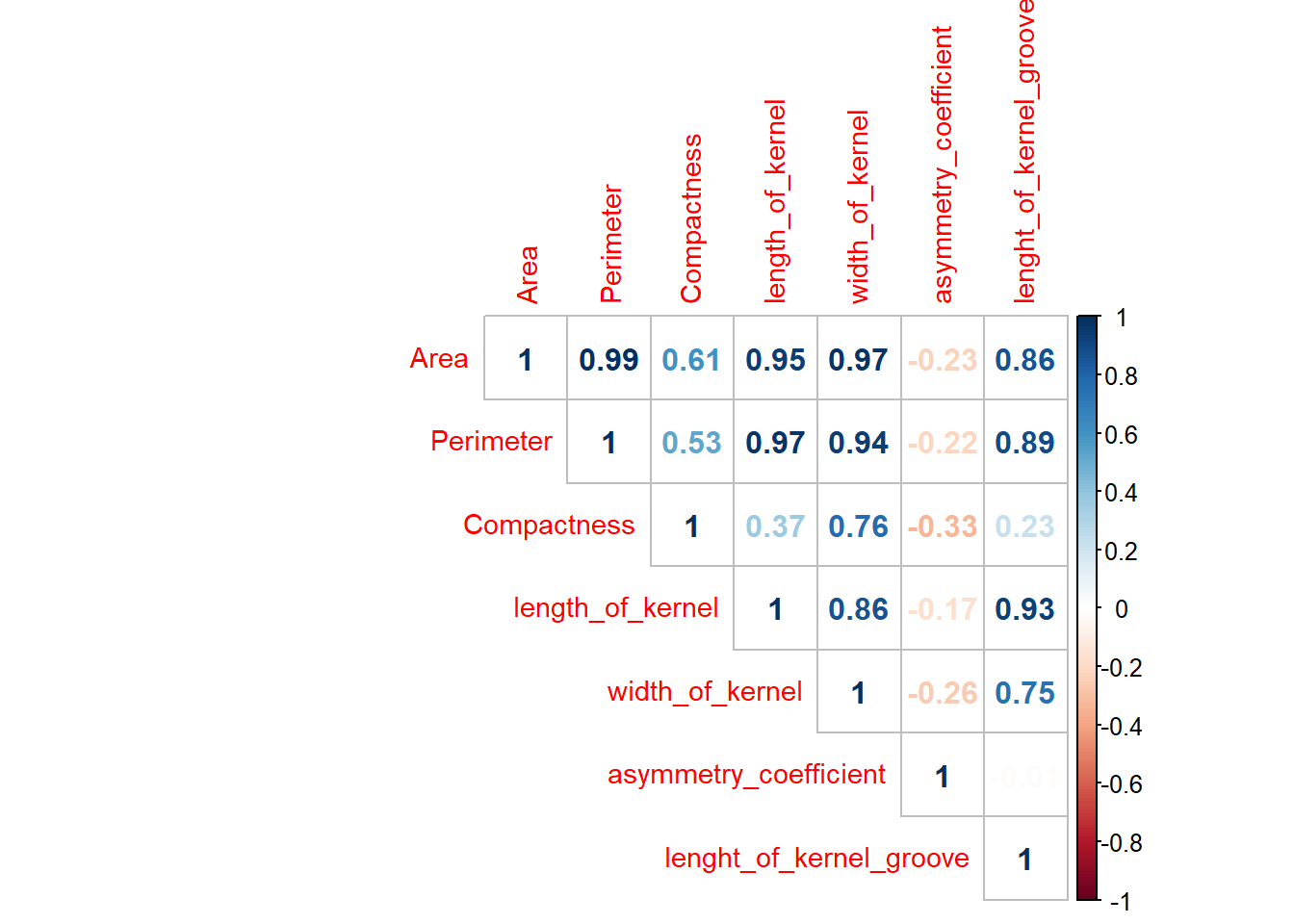Delete the variables

``````data\$Area<-NULL
data\$Perimeter<-NULL``````

It seems that everything ok, we can start with the analysis process.

## Clustering with K-Means

We obtain the mean silhouettes plot, which measures the separation distance between clusters (how close each point of a cluster is to points of neighboring clusters).

``plot(2:10,resultados[2:10],type="o",col="blue",pch=0,xlab="Numero de clusters",ylab="Silueta")``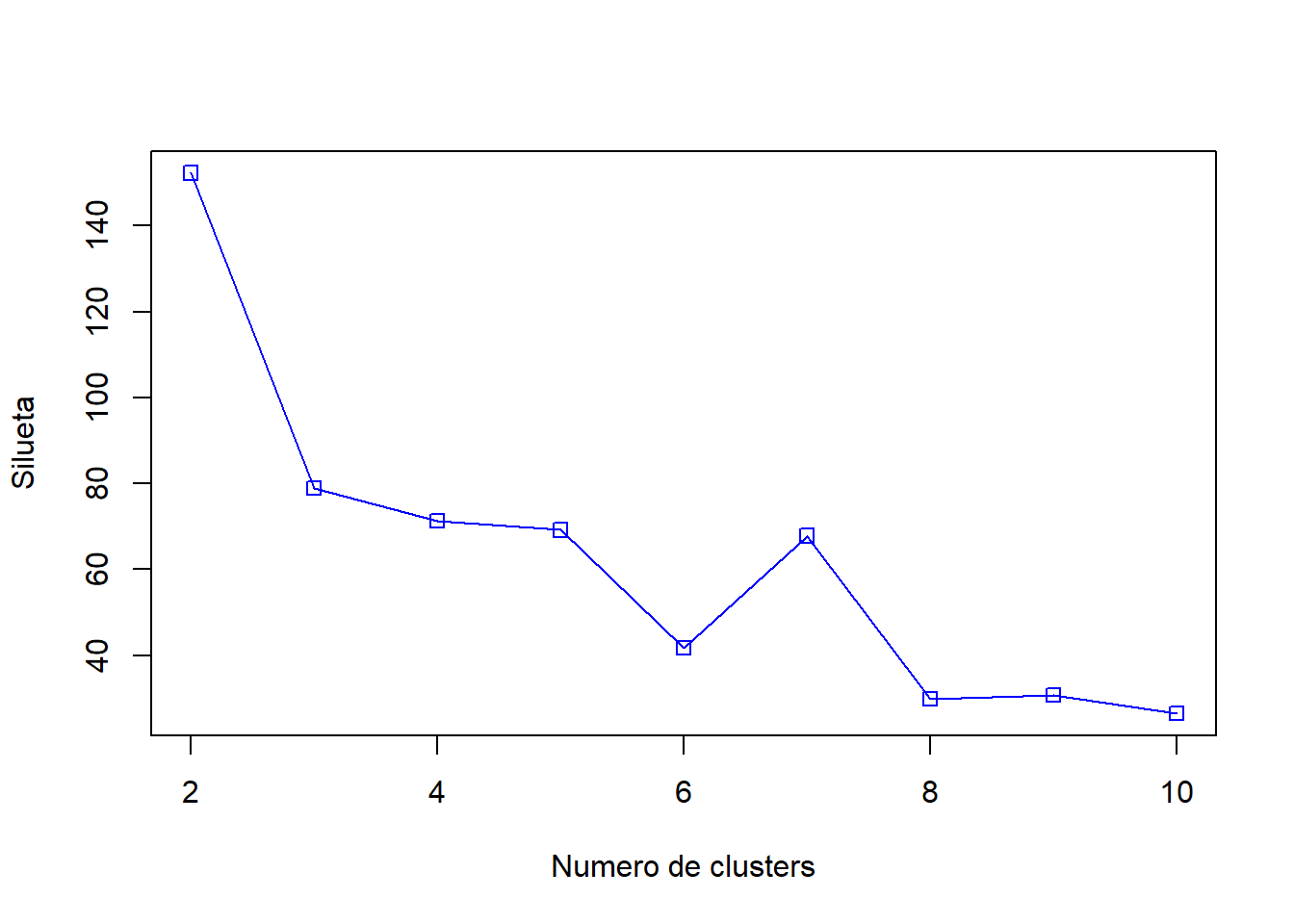It is see that the best number of clusters is 2.

We test with the best model, obtaining the sum of squares to define the intra-cluster variance.

``````d<-daisy(data)
for (i in c(2,3,4,5,6,7,8,9,10))
{
fit           <- kmeans(data, i)
y_cluster     <- fit\$cluster
sk            <- silhouette(y_cluster, d)
}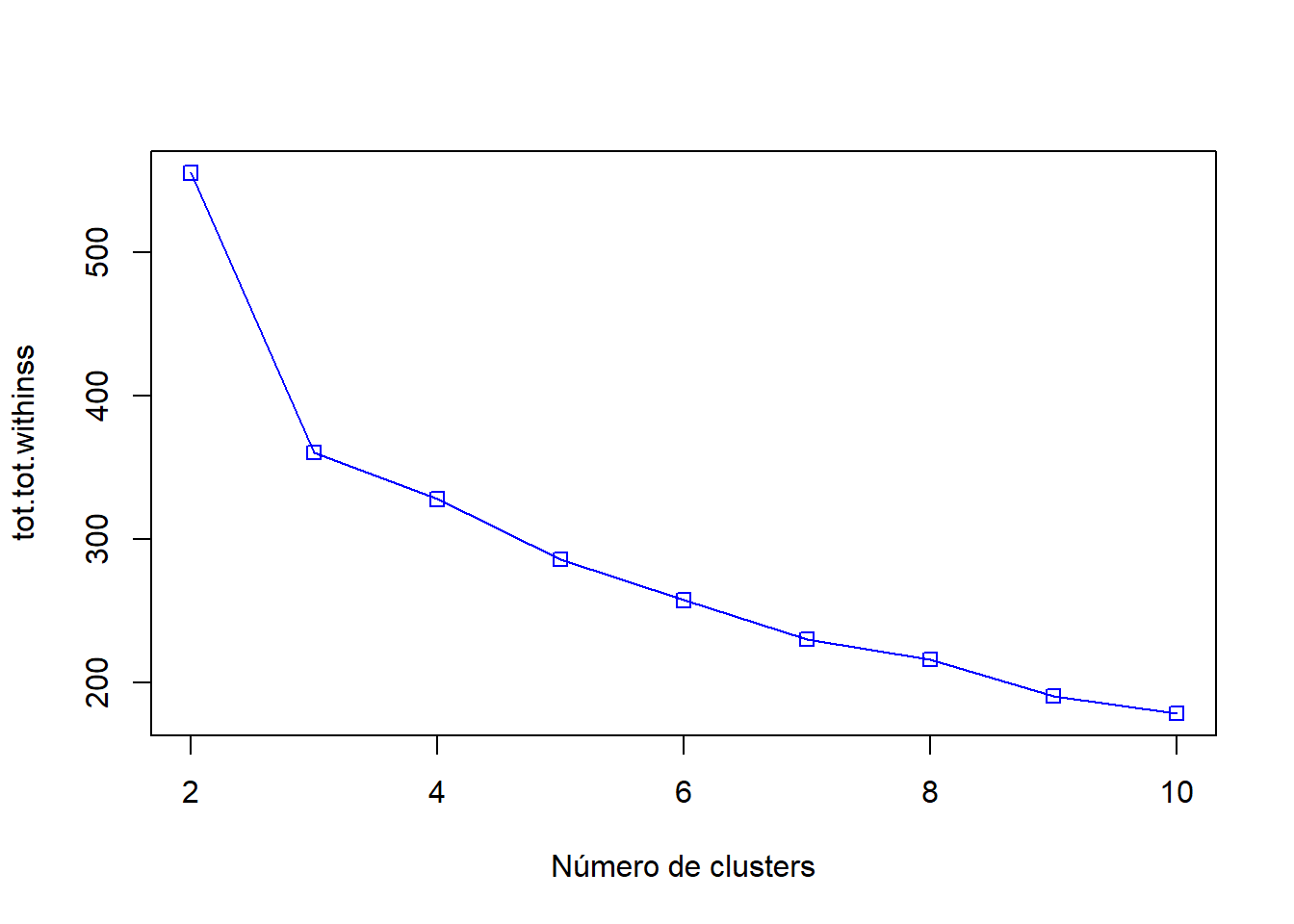In this case we can say that the most appropriate value is 3 which is where the bend of the curve is located.

We continue testing, with the function kmeansruns with the criteria asw (mean silhouette) and ch (Calinski-Harabasz), which estimates the ratio between the distance between clusters and the intra-cluster distance, the higher the ratio, the better the model.

``````library(fpc)
fit_ch  <- kmeansruns(data, krange = 1:10, criterion = "ch")
fit_asw <- kmeansruns(data, krange = 1:10, criterion = "asw") ``````

We obtain the 2 values

.
``fit_ch\$bestk``
``##  3``
``fit_asw\$bestk``
``##  2``

We see that the values are 3 Calinski-Harabasz for and 2 for the average silhouette, shown in the graphs.

``plot(1:10,fit_asw\$crit,type="o",col="blue",pch=0,xlab="Número de clústers",ylab="Criterio silueta media")``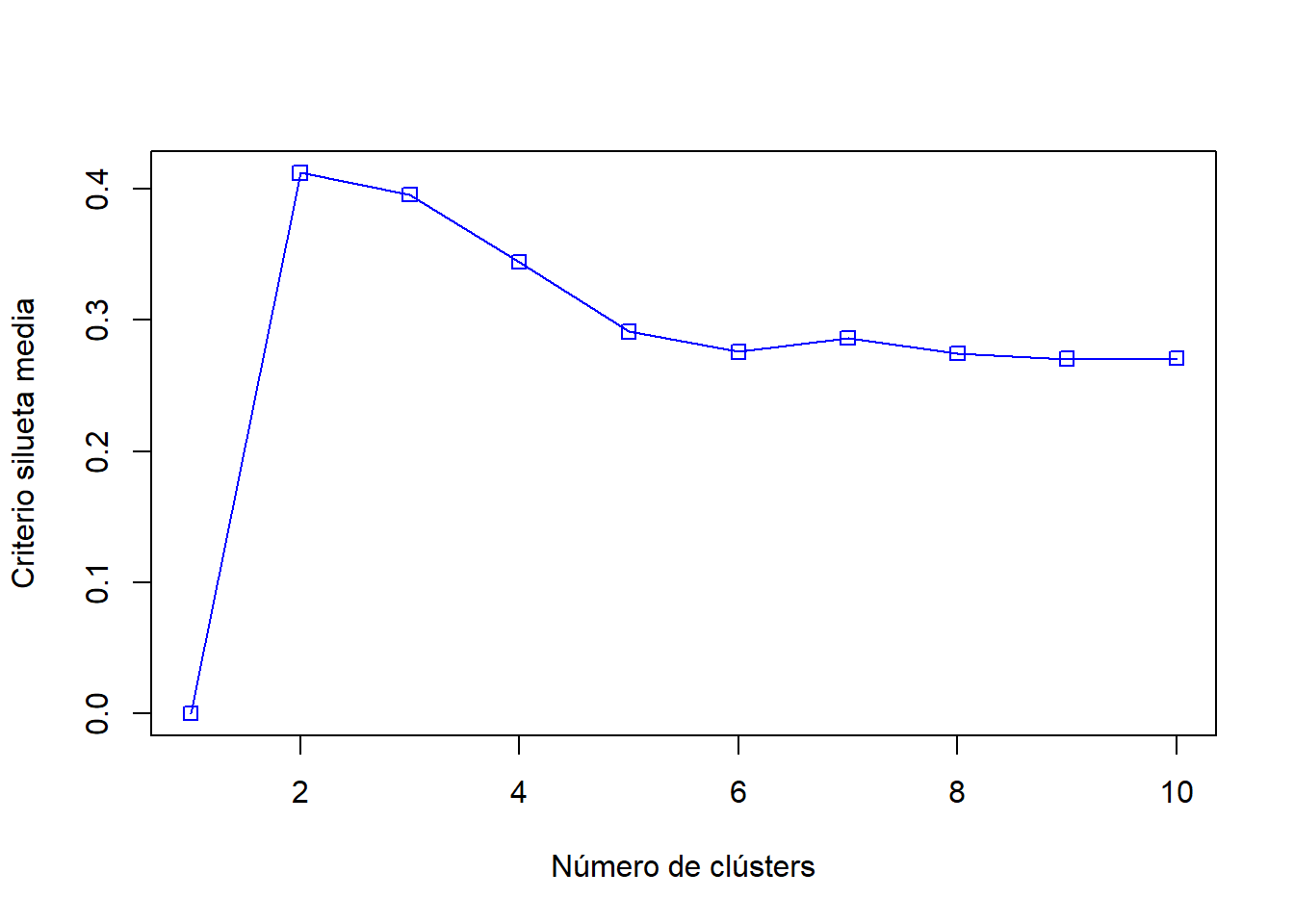``plot(1:10,fit_ch\$crit,type="o",col="blue",pch=0,xlab="Número de clústers",ylab="Criterio Calinski-Harabasz")``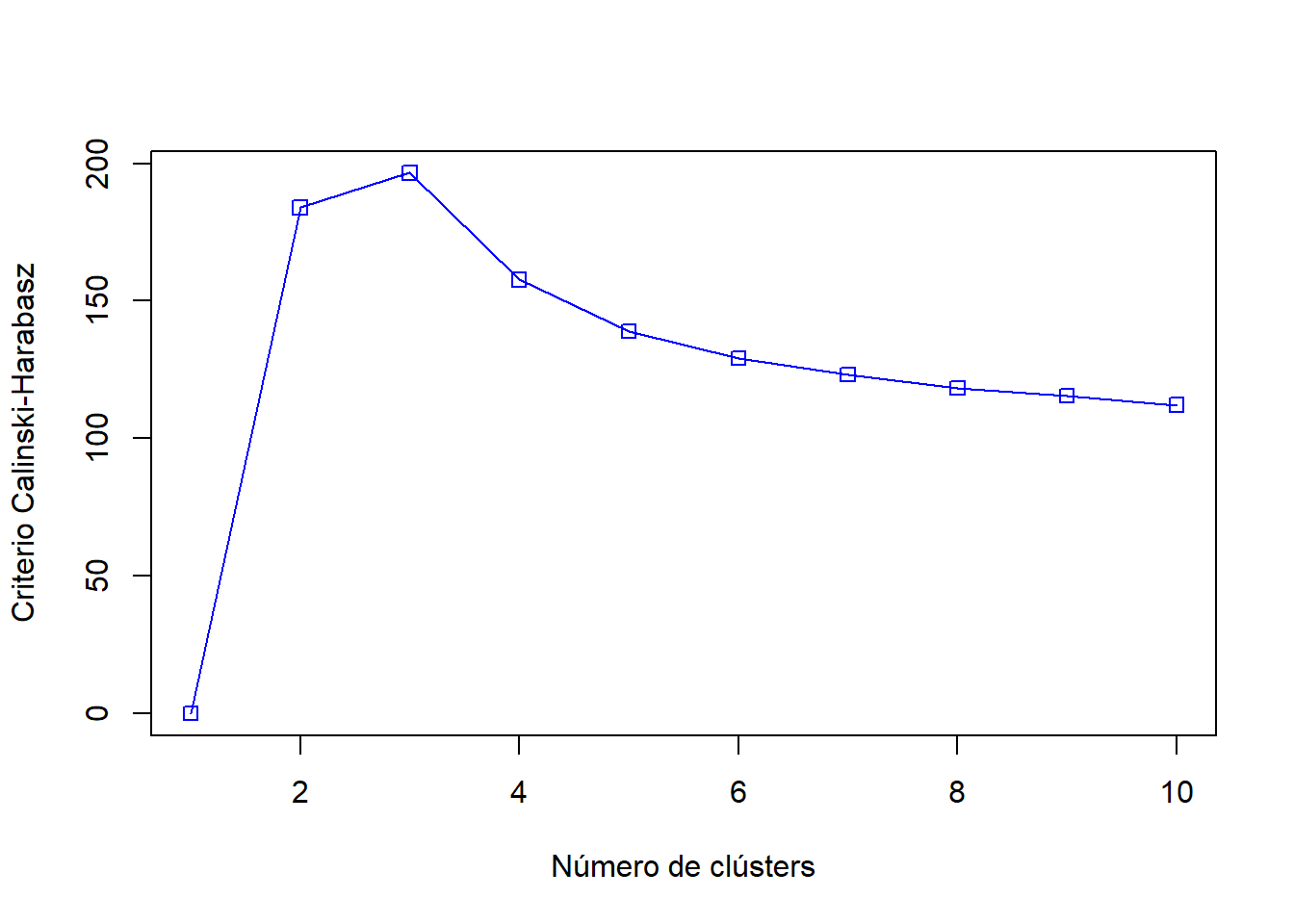Let's now see how k-means classifies with 3 classes, which are the classes into which it divides the original dataset.

We show the classification by pairs of variables.

``````kmeansclusters <- kmeans(data, 3)

# Kernel length and width
plot(data[c(2,3)], col=kmeansclusters\$cluster)``````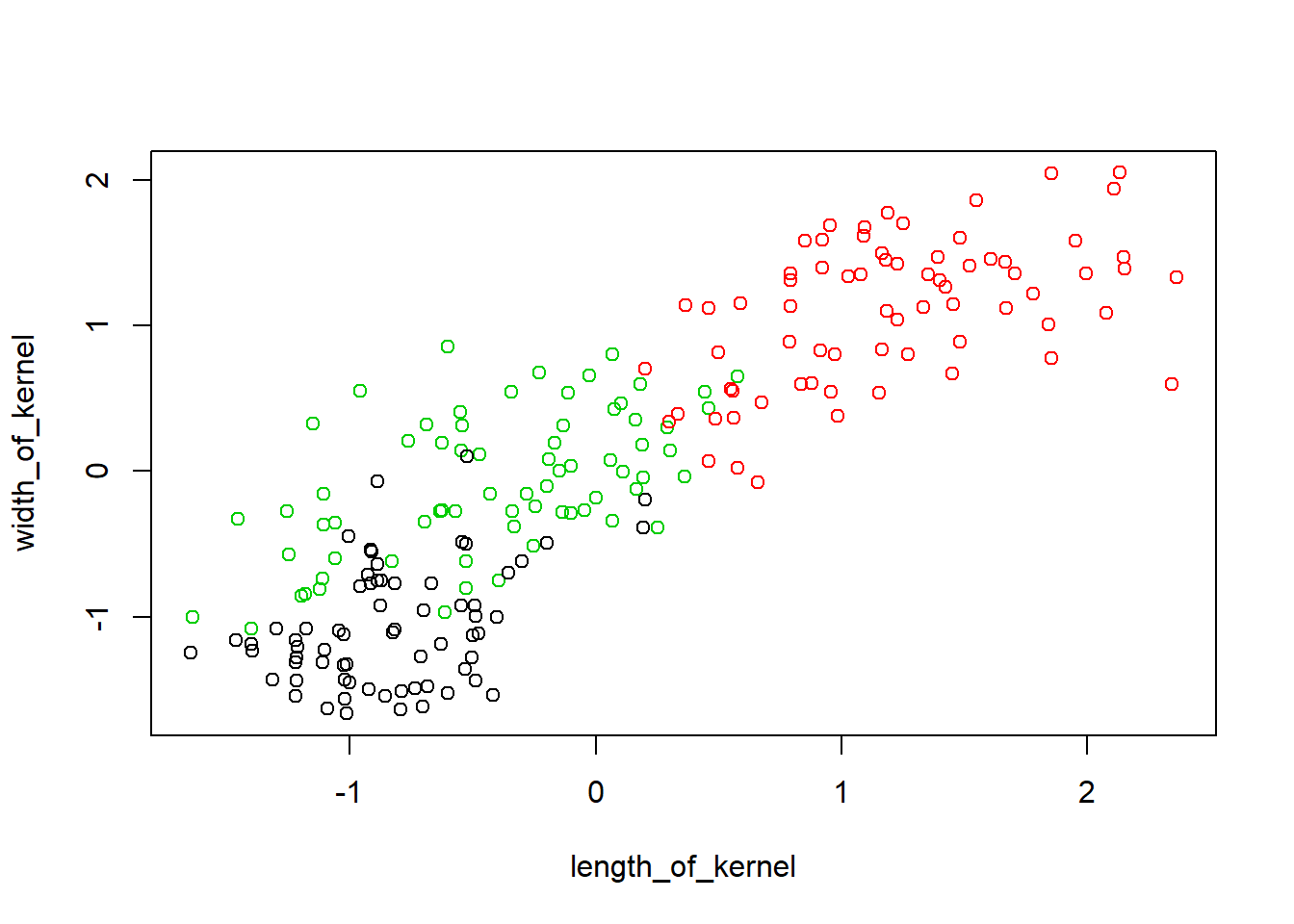As we can see the classification according to the length and width attributes shows the 3 differentiated groups, it seems a good classification criterion, although two groups appear somewhat mixed. We can also see it in the following graph where it shows the classes in original dataset.

``plot(data[c(2,3)], col=dataorig\$class)``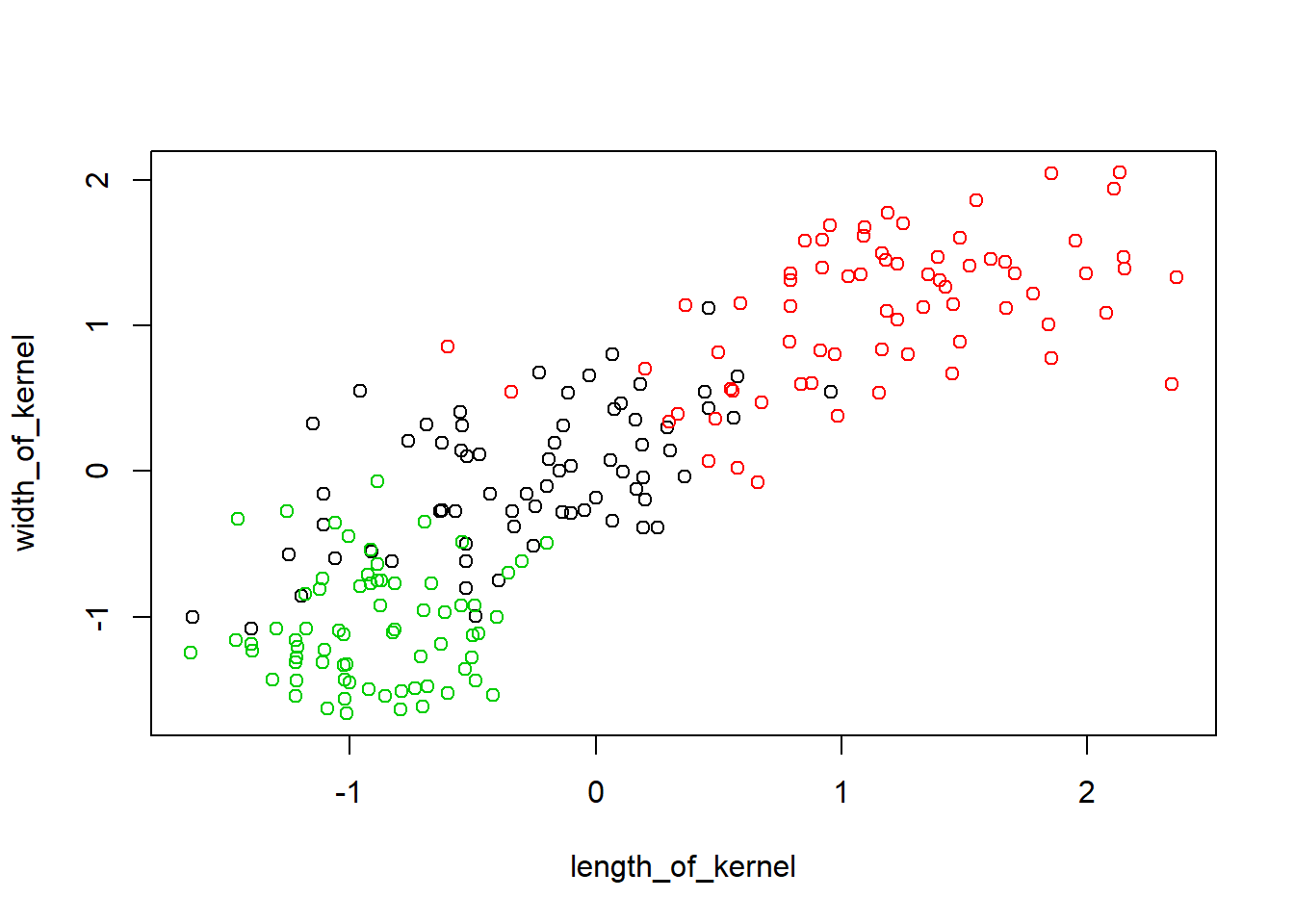Let's see the case of the coefficient of asymmetry with core length.

``plot(data[c(2,4)], col=kmeansclusters\$cluster)``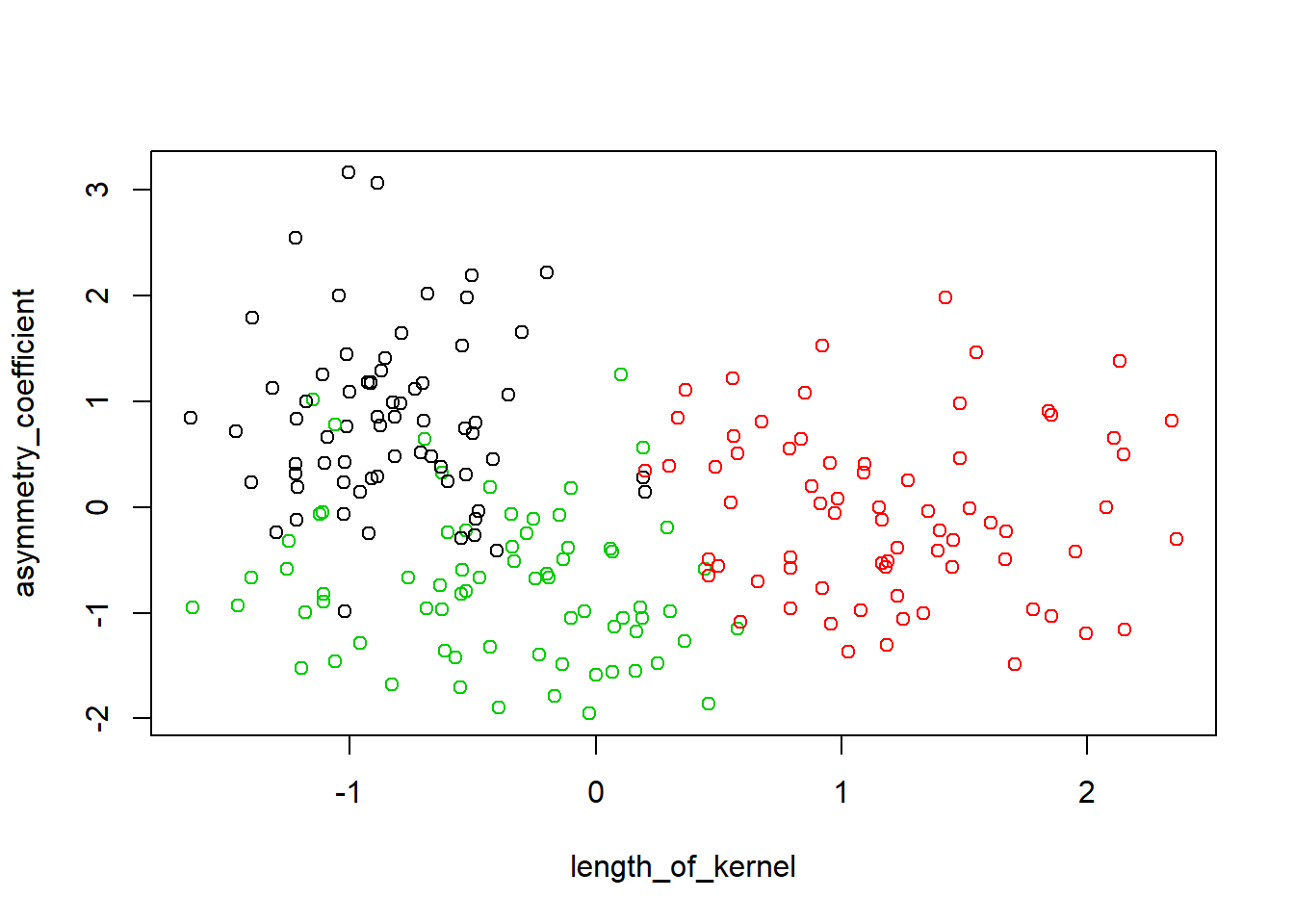``plot(data[c(2,4)], col=dataorig\$class)``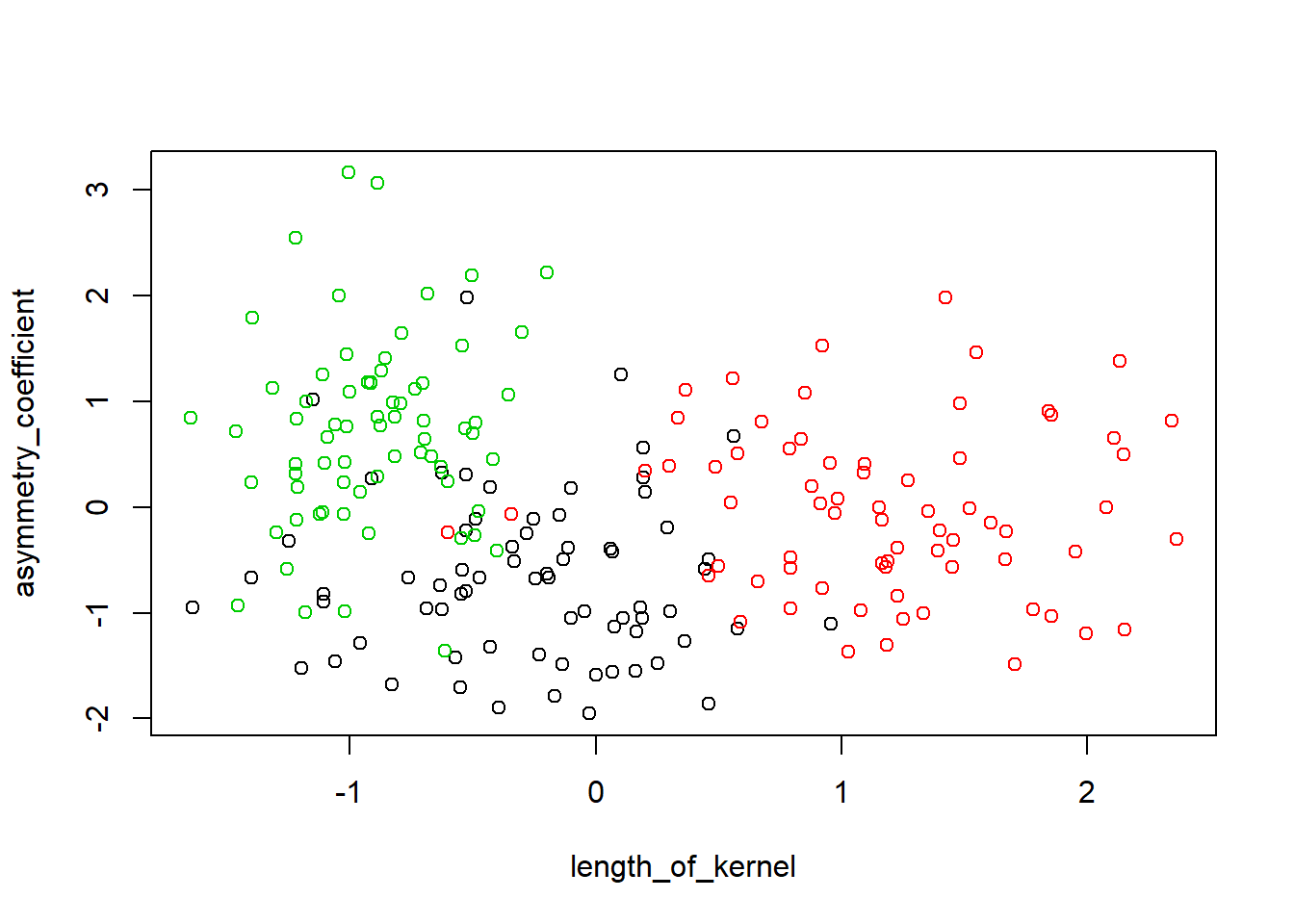We see the percentage of success.

``table(kmeansclusters\$cluster,dataorig\$class)``
``````##
##      1  2  3
##   1  6  0 62
##   2  3 68  0
##   3 61  2  8``````

Although the assigned group number is not the same, we the combinations that have the most data to be correct. We can see that we have a 90% correctness rate.

``````porc=100-19*100/188
porc``````
``##  89.89362``

To evaluate the model further we will obtain the quality with the Silhouette parameter that determines the cohesion of the clusters, measuring the similarity of each data with those of its cluster compared to the data of other clusters. We observe that there are no negative values, indicating that the clusters are well cohesive.

We will now inspect the clusters.

Let's also inspect the silhouette plots, we see few values below zero, which indicates good quality in the model.The mean silhouette value is also a good indicator, we will compare it with the rest of the models.

``````library(factoextra)
sil<-silhouette(kmeansclusters\$cluster,daisy(data))
fviz_silhouette(sil,label=FALSE,print.summary=FALSE)``````